# Voltage Analysis of Multilevel Diode Clamped Inverter with SVPWM Technique

Voltage Analysis of Multilevel Diode Clamped Inverter with SVPWM Technique

Ch. N. Narasimha Rao P. Siva Prasad G. Durga sukumar Y. Srinivasa Rao

Assistant Professor, EEE Department, VFSTR (Deemed to be University), Guntur, AP, India

Teaching Associate, L&T Edutech, Chennai, Tamilnadu, India

Professor, Department of EEE, Vignan Institute of Technology and Science, Hyderabad, India

Assistant Professor, EEE Department, VFSTR (Deemed to be University), Guntur, AP, India

Corresponding Author Email:
cherukuri.narsi@gmail.com
Page:
135-141
|
DOI:
https://doi.org/10.14447/jnmes.v25i2.a07
21 July 2021
|
Accepted:
2 June 2022
|
Published:
10 June 2022
| Citation

OPEN ACCESS

Abstract:

The quantity of direct current voltage steps that are needed by the inverter connect is characterized based on the quantity of levels in an inverter bridge to accomplish a specific electric potential at its output. The best technique for settling the voltages applied to the gadgets is by clipping therefore utilizing dc voltage sources or huge capacitors, which momentarily act as voltage sources. Multilevel topology dependent on specific guideline, the input voltages applied to the devices can be controlled and restricted. A benefit of multilevel inverters contrasted that the yield voltage spectra are altogether better performed. Henceforth, the yield potentials can be sifted with more modest responsive segments, and furthermore, the exchanging frequencies of the gadgets can be diminished. Two advantages with the capacity to manage higher voltage levels present on multilevel inverters is a vital job in the field of high quality produced wave form applications. In this paper, the three levels Diode-clamped inverter incorporates displaying, recreation, plan execution, and examination. Space Vector Balance will be utilized, to dispose of the basic mode electric potentials by exchanging between the various states.

Keywords:

SVM, NPC, Multilevel inverter

1. Introduction

Multilevel inverters have drawn in much interest from specialists particularly in implementing high electric potentials and huge engine drive applications. This expanded acknowledgment of staggered inverter is because of the limits of the customary two-level yield inverters in taking care of high force transformations. The multilevel inverters can be created Diode-Clipped multilevel Inverter Utilizing Space Vector Adjustment by either utilizing numerous three-stage spans or by expanding the quantity of exchanging gadgets per stage, to build the quantity of levels .

The idea of a staggered inverter includes using a variety of arrangement changing gadgets to play out the force transformation in a little increment of potential steps by blending the flight of stairs voltage from a few degrees of dc capacitor voltages.

Space vector regulation has been the balance procedure for the inverter as it empowers at power framework recurrence which for the most part creates in pulse width modulation as carrier frequency, makes the SVM more alluring to be utilized in excessive force and voltage applications .

2. Significance of Multi Level Inverters

A large portion of the examination works concerning multilevel control in exchanging of appliances in the inverters. Electric potentials lopsidedness which happens in the arrangement of assembling capacitor has been explored and a correlation investigation of each sort of multilevel inverters, for example, the Diode-Clipped, Flying Capacitor, and h-connect cascaded has additionally been performed and analyzed. Among all the multilevel inverters Diode Clinched inverter is proposed because of the huge harmonics energy put in the principle transporter part and the undoing of these segments between stage legs.

Staggered topologies depend on this guideline, and hence, the electric potentials applied to the appliances can be managed and restricted. A benefit of multilevel inverters contrasted and traditional two-level topology is that the yield potential spectra are fundamentally enhanced due to having more noteworthy accessibility of voltage levels. Consequently, the yield voltages can be sifted with more modest receptive parts, and furthermore, the exchanging frequencies of the devices can be diminished. These two benefits with the ability to oversee higher voltage levels present on staggered inverters a crucial occupation in the field of high power implementations -.

In this work, Three-Level Diode-Clamped inverter includes modelling, simulation, design implementation and performance analysis. Space Vector Balance will be utilized, to get freed the normal mode possibilities by exchanging among the various states.

3. Three Level Diode Clipped Inverter with SVPWM Technique

3.1 Three-level diode clamped-inverter

The neutral point clasped inverter so called as the diode clamped inverter was earliest utilized. in a three-level inverter in which the mid voltage level was characterized as the impartial point. Since the NPC inverter successfully duplicates the device potential level without need of exact voltage coordinating .

A three-level diode-clamped inverter is shown in Fig 3.1, incorporate of (m-1) capacitors on dc bus, 2(m-1) switching devices and 2(m-2) clamping diodes per phase. The dc-bus electric potential is split into three levels by two series-connected bulk capacitors, C1 and C2. The neutral point N noted in the middle of the two capacitors.

## 1.jpg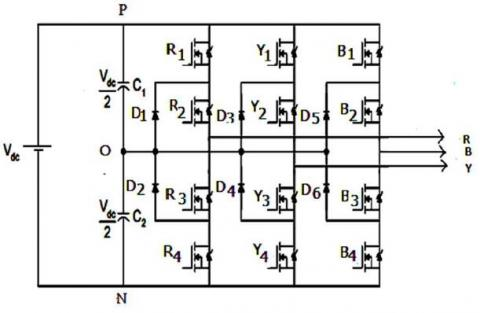Figure 3.1 Three-Level Diode-Clamped Inverter

Table 3.1 R phase switching status of diode clamped Inverter

 Switch status State Line Voltage Rl=ON, R2=ON, R3=OFF, R4=OFF, S=P Vrn=Vdc/2 Rl=OFF,  R2=ON, R3=ON,  R4=OFF S=O Vrn=0 Rl=OFF,  R2=OFF, R3=ON,  R4=ON S=N Vrn=-Vdc/2

3.2 Three-Level Space Vector Modulation Technique

Space Vector Modulation is a technique where the power converter generating the reference voltage is represented as a reference vector. There are 3 switching states for each inverter leg; P, O and N, for the operation of 3-level inverter. P represents the upper two switches ON while N denotes lower two switches are on with a terminal voltage of -Vdc/2. Exchanging state O connotes that the terminal potential reach zero. There exists an aggregate of 27 mixes of exchanging states for the diode-clipped inverter.

## 2.jpg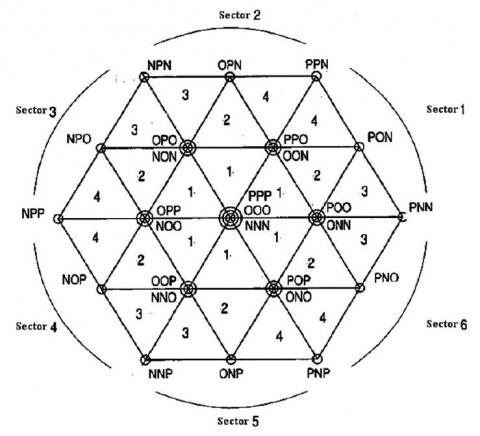Figure 3.2 Space vectors of three-level inverter with sector and region definition

The distinction between every one of the inverter expresses that produce a similar potential vector is standing out the heap is associated with the DC transport . Figure3.2 shows that the 27 inverter States can be characterized into five classifications.

a) All phases of the load are connected to the same potential. As a result, no net voltage is applied to the load. Corresponding to the states PPP, OOO and NNN.

b) Only the positive and negative rails of the dc bus are used. This represents to PNN, PPN, NPN, NPP, NNP and PNP.

c) In this case the load is connected to the positive rail, neutral point, and negative rail. Corresponding to PON, OPN, NPO, NOP, ONP and PNO.

d) The load is connected to the upper half of the bus only, causing capacitor C1 to discharge and C2 to charge. As a result the voltage of the neutral point starts to rise. Denoting to POO, PPO, OPO, OPP, OOP and POP.

e) It is opposite to case (d)

f)  The load is connected to the lower half of the bus only, causing C2 to discharge and C1 to charge. Therefore, the neutral point voltage starts to decrease. corresponds to ONN, OON, NON, NOO, NNO and ONO.

The duration of each voltage vectors obtained by vector calculations is the command voltage vectors approximately compute using three adjacent vectors lying in the principle of SVPWM technique.

VA=Vref cosωt                      (3.1)

VB=Vref cos(ωt-2π/3)                            (3.2)

VC = Vref cos(ωt-4π/3)                      (3.3)

The following equations are used to implement the space vector PWM.

$V_{\alpha}+i V_{\beta}=\frac{2}{3}\left(V_{A}+V_{B} e^{i \frac{2 \pi}{3}}+V_{C} e^{i \frac{4 \pi}{3}}\right)$                    (3.4)

$\left.V^{*}=\sqrt{(} V_{\alpha}^{2}+V_{\beta}^{2}\right)$                        (3.5)

The below represents the modulation ratio of three-phase three-level inverter

$m=\frac{V^{*}}{\frac{2}{3} V_{d c}}$                          (3.6)

V* defines the measure of the reference electric potential vector rotating with an angle speed of $\varpi$.

4. Voltage Simulation Results

The Simulation is performed utilizing a 1hp resistive load fed by a three-level MOSFET PWM inverter.

## 3.jpg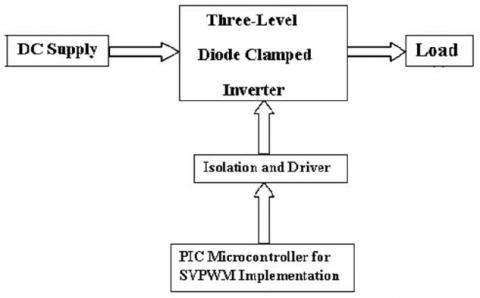Figure 4.1 Three-Level Inverter block diagram

4.1 Simulink model of three-level SVPWM inverter control circuit

## 4.jpg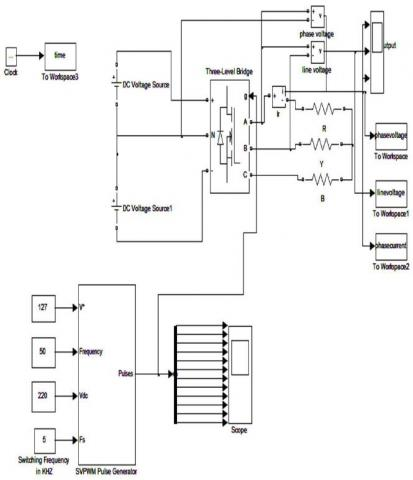Figure 4.2 Three Level SVPWM Inverter with control circuit

The SVPWM block has several internal blocks to produce pulses.

## 5.jpg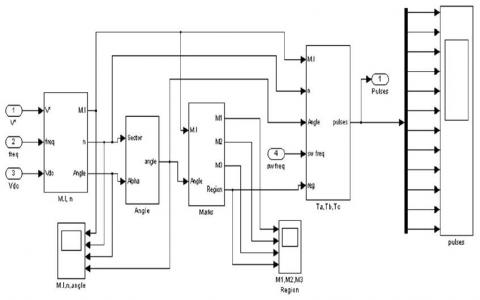Figure 4.3 Simulink model of control circuit

## 6.jpg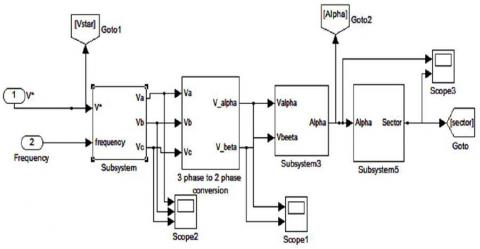Figure 4.4 Control circuit according to mathematical modeling

4.1.1 Reference Phase Voltages calculation

## 7.jpg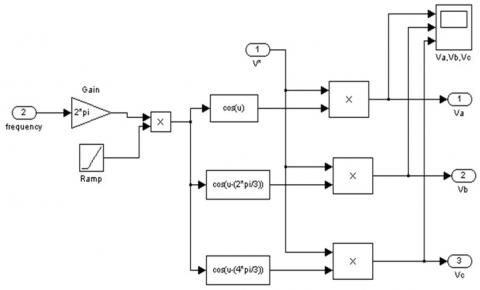Figure 4.5 Simulink model for calculation of reference phase voltages

## 8.jpg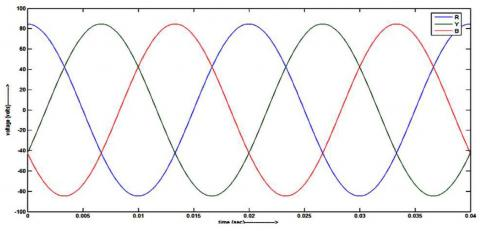Figure 4.6 Three phase voltages Va, Vb and Vc

4.1.2 Computation of $\mathbf{V}_{\alpha}, \mathbf{V}_{\beta}$:

## 9.jpg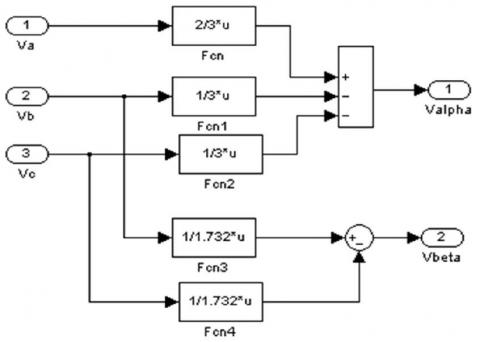Figure 4.7 Block for calculation of $\mathrm{V} \alpha, \mathrm{V} \beta$

## 10.jpg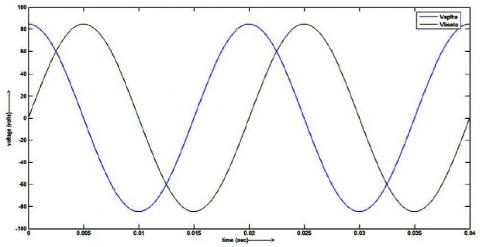Figure 4.8 Two phase voltages $\mathrm{V} \alpha, \mathrm{V} \beta$

4.1.3 Modulation Index (M.I)

Figures 4.9 and 4.10 show the block and determination of modulation index

## 11.jpg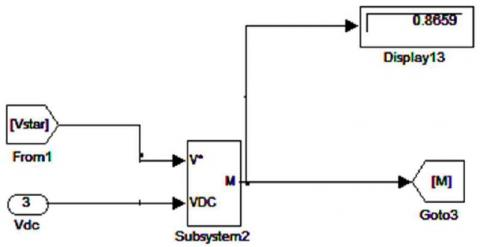Figure 4.9 Simulink model for calculation of M.I

## 12.jpg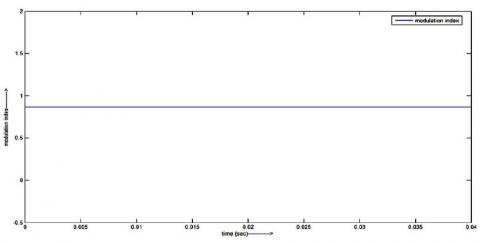Figure 4.10 Value of Modulation Index

4.1.4 Calculation of Voltage Space Vector Position Angle $(\alpha)$

## 13.jpg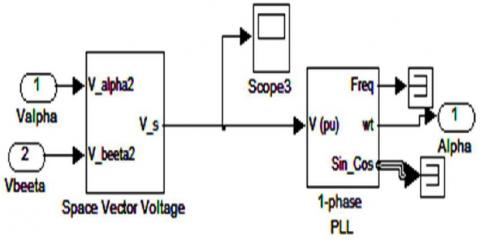Figure 4.11 Voltage Space Vector Position Angle

## 14.jpg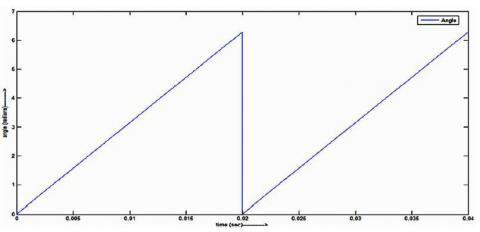Figure 4.12 Determination of angle

4.1.5 Determination of Time Durations Ta, Tb, Tc:

## 15.jpg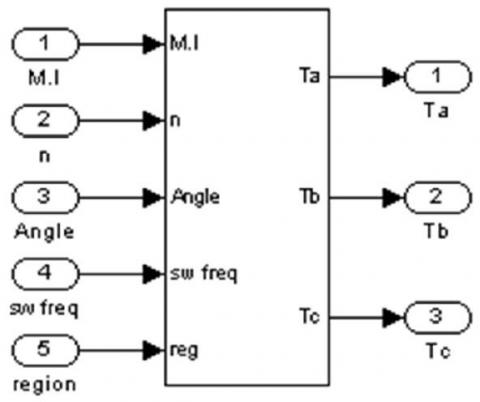Figure 4.13 Simulink Block to compute Ta, Tb, and Tc

4.1.6 Generation of PWM patterns of each MOSFET

Three level inverter has twelve switches which are MOSFETs.

## 16.jpg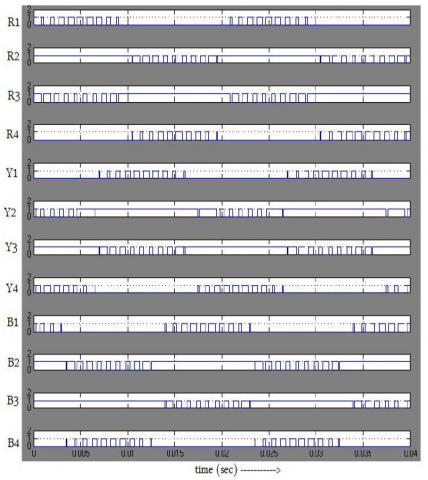Figure 4.14 Pulses for three level inverter

Figures 4.15, 4.16 and 4.17 show phase voltage, line voltage and line voltage from Three Level SVPWM inverter

## 17.jpg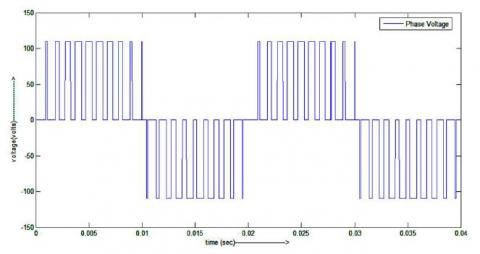Figure 4.15 Phase Voltage VRN

## 18.jpg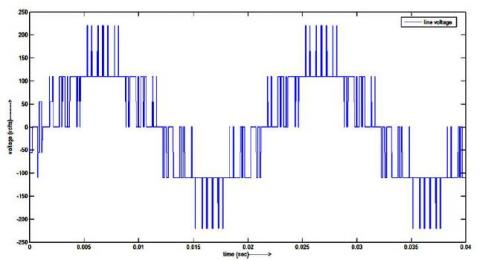Figure 4.16 Line Voltage VRB

## 19.jpg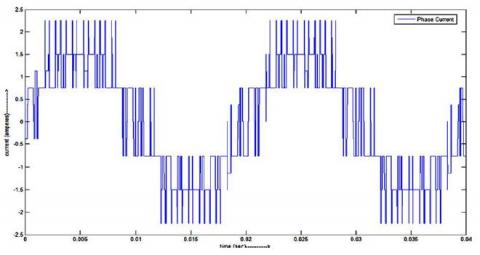Figure 4.17 Phase Current IR

5. Simulation Software and Hardware Implementation

5.1 Software implementation

A dedicated software program is developed in MPLAB platform. It is verified successfully in PROTEUS package which is specially made for micro controller based application. Figure 5.1 shows the pictorial representation of verified program in PROTEUS package. During the simulation 5V dc source is connected to pin 1.

5.1.1 Development of firing pulses using PIC18F452 microcontroller

## 20.jpgFigure 5.1 controlling circuit in PROTEUS platform

5.1.2 Output waveform

## 21.jpg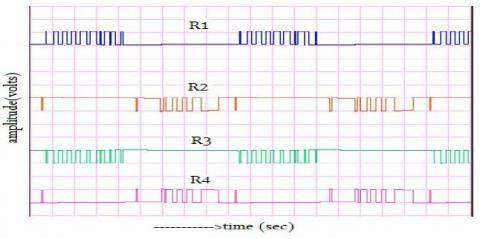Figure 5.2 Output from port RC0-RC3

## 22.jpg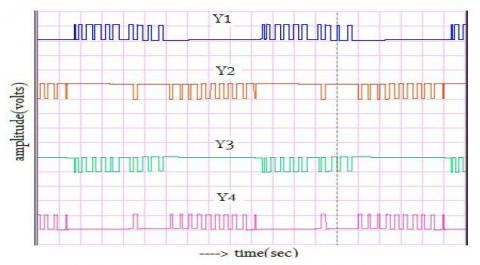Figure 5.3 Output from port RC4-RC7

## 23.jpg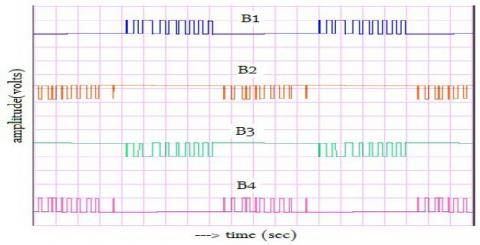Figure 5.4 Output from port RD0-RD3

5.2 Hardware implementation

Figure 5.5 shows the laboratory setup of the Three Level Diode Clamped multilevel inverter.

## 24.jpg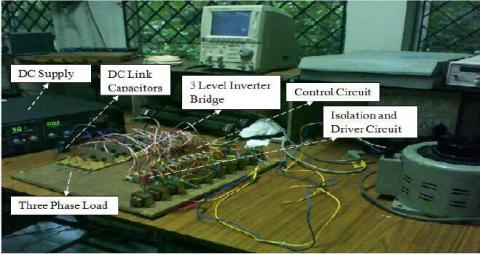Figure 5.5 Three-Level Inverter Hardware Prototype

5.3 Experimental results

Hardware parameters are shown in below table.

 S.NO Parameter value 1 DC Supply Voltage Vs 50V 2 DC Link Capacitor C1 = C2 2200µF, 220V 3 Switching Device MOSFET IRF740 4 Switching Frequency fs 5kHz 5 Output Frequency f 50Hz 6 Balanced-Y-Connected Resistive Load R 50 Ω/ph

Pulse from port RC0 Microcontroller is shown in Figure 5.14 with pulse width of 2ms/div and 5V/div amplitude

## 25.jpg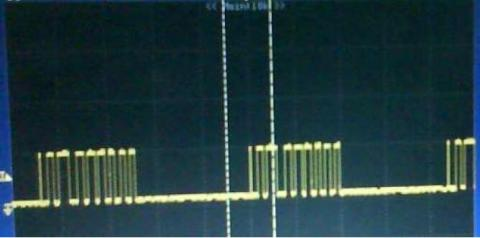Figure 5.6 output of port RCO (PWM of R1)

Figure 5.7 shows the firing pulse from both PIC microcontroller and opto-isolator with pulse width of 2ms and 5V amplitude.

## 26.jpg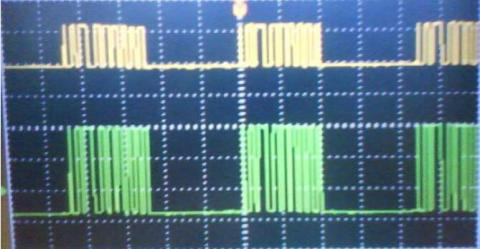Figure 5.7 Amplified pulses from MCT2E (PWM of R1)

Figure 5.8 shows the applied dc supply with time division of 2ms/div and amplitude of 10v/div.

## 27.jpg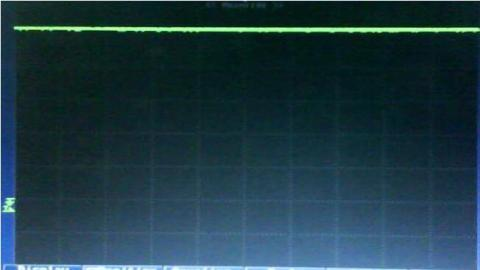Figure 5.8 DC supply applied to Inverter Bridge

Figure 5.9 shows the balanced voltage across one of the series capacitors, connected across the supply with time division of 2ms/div and amplitude of 10v/div.

## 28.jpg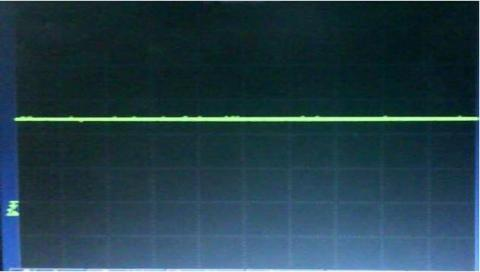Figure 5.9 Voltage across DC link Capacitor

Figures  5.10  and  5.11  show  phase  voltage VRN  and  line voltage VRB with time division of 2ms/div and amplitude of 10v/div.

## 29.jpg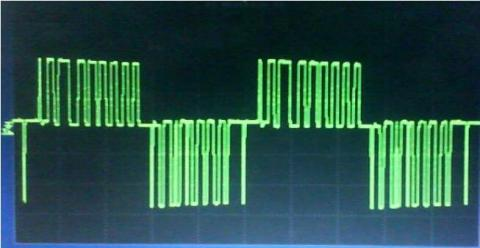Figure 5.10 Phase Voltage (VRN)

## 30.jpg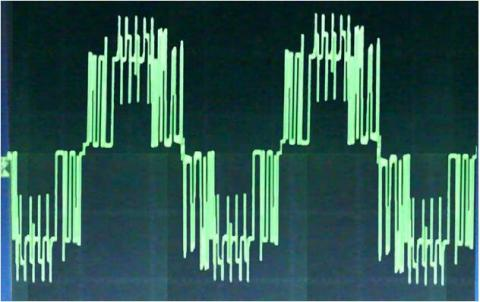Figure 5.11 Line Voltage (VRB)

6. Conclusion

The space vector pulse width modulation calculation for a three-level Unbiased Point clamped inverter was simulated and the results show the viability of NPC three-level inverter and fit with the hypothetical analysis well. The results have been given for a fair three-stage resistive burden with a 5 kHz exchanging frequency. Control signals for switching the power MOSFET’s have been generated using PIC18F452 microcontroller. MPLAB IDE 8.30 is used to program the PIC microcontroller and this program is verified using PROTEOUS software package. A three phase balanced resistive load is fed from this NPC inverter and the phase potentials and line voltages have been noticed. This three level NPC inverter is designed and fabricated in laboratory.

References

 Jae Hyeong Seo, Chang Ho Choi and Dong Seok Hyun, “A New Simplified Space Vector PWM method for Three-Level Inverters,” IEEE Transaction on Power Electronics vol.16no.4, Jul 2001, pp 545-550.

 Yadong LIU, Xuezhi Wu, Lipei Huang, “Implementation of Three-Inverter Using a Novel Space Vector Modulation Algorithm,” IEEE 2002.

 D.Rathnakumar and J.Lakshmana Perumal, T.Srinivasan, ”A New Software Implementation of Space Vector PWM,” IEEE Transaction on Power Electronics vol.18.NO.2, Mar 2005, pp 131-136.

 Chaturvedi, P., S. Jain and P. Agrawal, “Modelling, Simulation and Analysis of Three-Level Neutral Point Clamped Inverter using Matlab/Simulink/Power System Blockset”, Proceedings of the 8th International Conference on Electrical Machines and Systems 2005, Nanjing, China, Volume 2, 27-29 September 2005, pp. 1223-1227.

 Mouton, H.T., “Natural Balancing of Three-Level Neutral-Point-Clamped PWM Inverters”, IEEE Transaction on Industrial Electronics, Volume 49, Issue 5, October 2002, pp. 1017-1025.

 Peng, F.Z., “A Generalized Multilevel Inverter Topology with Self Voltage Balancing”, IEEE Industry Applications Conference 2000, Rome, Italy, Volume 3, 8-12 October 2000, pp. 2024-2031.

 Lei Lin, Yunping Zou, Jie Zhang, Xudong Zou, “Digital Implementation of Diode clamped Three Phase Three level SVPWM Inverter”, IEEE Transactions on Power Electronics, 2003 pp. 1413- 1417.